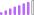cancel
Showing results for
Did you mean:

Fabric is Generally Available. Browse Fabric Presentations. Work towards your Fabric certification with the Cloud Skills Challenge.Post Partisan

## Return Value based on Last Date and Return Value Based on Second to Last Date (Penultimate Date)

Hi there,

This might be quite a simple one - sorry that I am new to DAX and learning. I have the following table (simplified)

 Asset Date Value aaa 24/07/2020 20 aaa aaa 25/07/2022 30 aaa 01/04/2019 50 aaa 04/06/2019 40 bbb 17/03/2021 70 bbb 10/02/2019 50 bbb bbb 14/03/2020 40 bbb 11/02/2018 80 bbb 10/02/2017 90 ccc 02/02/2019 30 ccc 02/02/2018 30

I am trying to create a table in my report that will reference this table to make it look like this:

 Asset Current Value Previous Value % Difference aaa 30 20 bbb 70 40 ccc 30 30

Basically I would like the asset listed 'distinctly', and then for current value column:

- each decided by finding the most recent date, and returning the value

For previous value

- find the second to last most recent date and return the value

For % difference

- The % difference between the above two

Any help really appreciated.

1 ACCEPTED SOLUTIONResolver I

It seems I forgot to include the SUM() function here.

Try ->
VAR ValueSecondLastDate = Calculate(SUM(Table1[Value]), Table1[Date] = SecondLastDate)

When writing measures, you need to use functions on the columns

5 REPLIES 5Resolver I

Edit to Above Formula ->

Try the following ->

ValueLastDate = Calculate(SUM(Table1[Value]), LASTDATE(Table1[Date]))

ValueSecondLastDate =

VAR SecondLastDate = CALCULATE
(MAX(Table1[Date]), Table1[Date] < MAX(Table1[Date]))

VAR ValueSecondLastDate = Calculate(Table1[Value], Table1[Date] = SecondLastDate)

RETURN
ValueSecondLastDate

Value Delta % =
VAR Delta = ValueLastDate - ValueSecondLastDatee
Return
Calculate(Divide(Delta, ValueSecondLastDate))Post Partisan

Many thanks for this! I have split into two measures. Measure 1:

ValueLastDate = Calculate(SUM(Table1[Value]), LASTDATE(Table1[Date]))

This works fine.

But measure 2:

ValueSecondLastDate =

VAR SecondLastDate = CALCULATE
(MAX(Table1[Date]), Table1[Date] < MAX(Table1[Date]))

VAR ValueSecondLastDate = Calculate(Table1[Value], Table1[Date] = SecondLastDate)

RETURN
ValueSecondLastDate

This is giving me an error - Cannot find table 'Table1[Value]'. And the intellisense when typing only provides other DAX measures instead of any table and column reference.
What am I doing wrong?Resolver I

It seems I forgot to include the SUM() function here.

Try ->
VAR ValueSecondLastDate = Calculate(SUM(Table1[Value]), Table1[Date] = SecondLastDate)

When writing measures, you need to use functions on the columnsPost Partisan

Thank you so much @arichard19 for you help. Works perfectly. I just added the square brackets for the references to other measures where needed in the DAXResolver I

Try the following ->

LastDate = Calculate(SUM(Table1[Value]), LASTDATE(Table1[Date]))

SecondLastDate =
VAR LastDate = LASTDATE(Table1[Date]))
RETURN
Calculate(SUM(Table1[Value]), MAX(Table1[Date]) < LastDate))

Delta % =
VAR Delta = LastDate - SecondLastDate
Return
Calculate(Divide(Delta, SecondLastDate))Announcements#### Power BI Monthly Update - November 2023

Check out the November 2023 Power BI update to learn about new features.#### Fabric Community News unified experience

Read the latest Fabric Community announcements, including updates on Power BI, Synapse, Data Factory and Data Activator.#### The largest Power BI and Fabric virtual conference

130+ sessions, 130+ speakers, Product managers, MVPs, and experts. All about Power BI and Fabric. Attend online or watch the recordings.Top Solution Authors
Top Kudoed Authors
Users online (2,717)